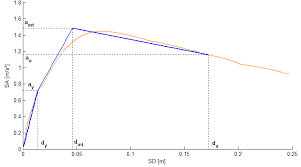## How to Calculate and Solve for Structure Capacity | Water BudgetThe image above represents structure capacity.

To compute for structure capacity, three essential parameters are needed and these parameters are Entrance Factor (C), Crest Length (L) and Depth of Flow (H).

The formula for calculating the structure capacity:

Q = CLH1.5

Where:

Q = Structure Capacity
C = Entrance Factor
L = Crest Length
H = Depth of Flow

Let’s solve an example;
Find the structure capacity when the entrance factor is 6, the crest length is 10 and the depth of flow is 12.

This implies that;

C = Entrance Factor = 6
L = Crest Length = 10
H = Depth of Flow = 12

Q = CLH1.5
Q = (6)(10)(12)1.5
Q = (6)(10)(41.56)
Q = 2494.15

Therefore, the structure capacity is 2494.15.

Calculating the Entrance Factor when the Structure Capacity, the Crest Length and the Depth of Flow is Given.

C = Q / LH1.5

Where;

C = Entrance Factor
Q = Structure Capacity
L = Crest Length
H = Depth of Flow

Let’s solve an example;
Find the entrance factor when the structure capacity is 20, the crest length is 8 and the depth of flow is 4.

This implies that;

Q = Structure Capacity = 20
L = Crest Length = 8
H = Depth of Flow = 4

C = Q / LH1.5
C = 20 / (8)(8)
C = 20 / 64
C = 0.3125

Therefore, the entrance factor is 0.3125.